# NCERT Solutions for Class 8 Maths Chapter 6 Squares and Square Roots| PDF Download

Here you will get Chapter 6 Squares and Square Roots Classs 8 Maths NCERT Solutions which will make learning exciting and cover the syllabus in less time. You can download PDF of NCERT Solutions for Class 8 Maths Chapter 6 which will serve as beneficial tool that can be used to recall various questions any time. NCERT Solutions is a good way through which one can build their basic knowledge.

Through the help of NCERT Solutions for Class 8 Maths will be useful in the preparation of examinations and make you aware of concepts of the chapter. These NCERT Solutions  are updated according to the latest NCERT Class 8 Maths textbook and syllabus.Page No: 96

Exercise 6.1

1. What will be the unit digit of the squares of the following numbers?
(i) 81
(ii) 272
(iii) 799
(iv) 3853
(v) 1234
(vi) 26387
(vii) 52698
(viii) 99880
(ix) 12796
(x) 55555

Solution

The unit digit of square of a number having 'a' at its unit place ends with a×a.

(i) The unit digit of the square of a number having digit 1 as unit’s place is 1.
∴ Unit digit of the square of number 81 is equal to 1.

(ii) The unit digit of the square of a number having digit 2 as unit’s place is 4.
∴ Unit digit of the square of number 272 is equal to 4.

(iii) The unit digit of the square of a number having digit 9 as unit’s place is 1.
∴ Unit digit of the square of number 799 is equal to 1.

(iv) The unit digit of the square of a number having digit 3 as unit’s place is 9.
∴ Unit digit of the square of number 3853 is equal to 9.

(v) The unit digit of the square of a number having digit 4 as unit’s place is 6.
∴ Unit digit of the square of number 1234 is equal to 6.

(vi) The unit digit of the square of a number having digit 7 as unit’s place is 9.
∴ Unit digit of the square of number 26387 is equal to 9.

(vii) The unit digit of the square of a number having digit 8 as unit’s place is 4.
∴ Unit digit of the square of number 52698 is equal to 4.

(viii) The unit digit of the square of a number having digit 0 as unit’s place is 01.
∴ Unit digit of the square of number 99880 is equal to 0.

(ix) The unit digit of the square of a number having digit 6 as unit’s place is 6.
∴ Unit digit of the square of number 12796 is equal to 6.

(x) The unit digit of the square of a number having digit 5 as unit’s place is 5.
∴ Unit digit of the square of number 55555 is equal to 5.

2. The following numbers are obviously not perfect squares. Give reason.
(i) 1057
(ii) 23453
(iii) 7928
(iv) 222222
(v) 64000
(vi) 89722
(vii) 222000
(viii) 505050

Solution

As we know that natural numbers ending in the digits 0, 2, 3, 7 and 8 are not perfect square.
∴ 1057, 23453, 7928, 222222, 64000, 89722, 222000 and 505050 are not perfect squares.

3. The following numbers are obviously not perfect squares. Give reason.

Solution

As we know that the square of an odd number is odd and the square of an even number is even. Therefore,
(i) The square of 431 is an odd number.
(ii) The square of 2826 is an even number.
(iii) The square of 7779 is an odd number.
(iv)The square of 82004 is an even number.

4.The squares of which of the following would be odd numbers?
112 = 121
1012 = 10201
10012 = 1002001
1000012 = 1 .......2.........1
100000012 = ..........................

Solution

We observe that the square on the number on R.H.S of the equality has an odd number of digits such that the first and last digits both are 1 and middle digit is 2. And the number of zeros between left most digits 1 and the middle digit 2 and right most digit 1 and the middle digit 2 is same as the number of zeros in the given number.
∴ 100001= 10000200001
100000012 = 100000020000001

5. Observe the following pattern and supply the missing numbers.
112 = 121
1012 = 10201
101012 = 102030201
10101012 = ...........................
............2  = 10203040504030201

Solution

We observe that the square on the number on R.H.S of the equality has an odd number of digits such that the first and last digits both are 1. And, the square is symmetric about the middle digit. If the middle digit is 4, then the number to be squared is 10101 and its square is 102030201.
So, 1010101=1020304030201
1010101012 =10203040505030201

6. Using the given pattern, find the missing numbers.
12 + 22 + 22 = 32
22  + 32 + 62 = 72
32  + 42 + 122 = 132
42  + 52 + _ = 212
5  + _ 2 + 302 = 312
6  + 7 + _ 2 = __2

Solution

Given, 12  + 22 + 2= 32
i.e  1+ 2+ (1×2 )= ( 1+ 2-1 × 2 )2

• 2+ 3+ 6=72
∴ 2+ 3+ (2×3 )= (2+ 3-2 × 3)

• 3+ 4+ 12= 132
∴ 3+ 4+ (3×4 )= (3+ 4- 3 × 4)

• 4+ 5+ (4×5 )= (4 + 5- 4 × 5)
∴ 4+ 5+ 20= 212

• 5+ 6+ (5×6 )= (52+ 6- 5 × 6)2
∴ 5+ 6 + 30 = 312

• 6+ 7+ (6×7 )= (6+ 7- 6 × 7)
∴ 6+ 72 + 42= 432

7. Without adding, find the sum.
(i) 1 + 3 + 5 + 7 + 9

Solution

Sum of first five odd number = (5)= 25

(ii) 1 + 3 + 5 + 7 + 9 + I1 + 13 + 15 + 17 +19

Solution

Sum of first ten odd number = (10)= 100

(iii) 1 + 3 + 5 + 7 + 9 + 11 + 13 + 15 + 17 + 19 + 21 + 23

Solution

Sum of first thirteen odd number = (12)= 144

8. (i) Express 49 as the sum of 7 odd numbers.

Solution

We know, sum of first n odd natural numbers is n.
Since, 49 = 7
∴ 49 = sum of first 7 odd natural numbers = 1 + 3 + 5 + 7 + 9 + 11 + 13

(ii) Express 121 as the sum of 11 odd numbers.

Solution

Since, 121 = 112
∴ 121 = sum of first 11 odd natural numbers = 1 + 3 + 5 + 7 + 9 + 11 + 13 + 15 + 17 + 19 + 21

9. How many numbers lie between squares of the following numbers?
(i) 12 and 13
(ii) 25 and 26
(iii) 99 and 100

Solution

Between n2 and (n+1)2, there are 2n non–perfect square numbers.
(i) 12and 132 there are 2×12 = 24 natural numbers.
(ii) 252 and 262 there are 2×25 = 50 natural numbers.
(iii) 992 and 100 there are 2×99 =198 natural numbers.

Exercise 6.2

1. Find the square of the following numbers.
(i) 32
(ii) 35
(iii) 86
(iv) 93
(v) 71
(vi) 46

Solution

(i) (32)2
= (30 +2)2
= (30)+ (2)+ 2×30×2 [Since, (a+b)= a2+b2 +2ab]
= 900 + 4 + 120
= 1024

(ii) (35)2
= (30+5 )2
= (30)+ (5)+ 2×30×5 [Since, (a+b)= a2+b+2ab]
= 900 + 25 + 300
= 1225

(iii) (86)2
= (90 - 4)2
= (90)+ (4)- 2×90×4 [Since, (a-b)= a+ b- 2ab]
= 8100 + 16 - 720
= 8116 - 720
= 7396

(iv)  (93)2
= (90+3 )2
= (90)+ (3)+ 2×90×3 [Since, (a+b)= a2+b+2ab]
= 8100 + 9 + 540
= 8649

(v) (71)2
= (70+1 )2
= (70)+ (1)+2×70×1 [Since, (a+b)= a2+b+2ab]
= 4900 + 1 + 140
= 5041

(v) (46)2
= (50 -4 )2
= (50)+ (4)- 2×50×4 [Since, (a-b)= a+ b- 2ab]
= 2500 + 16 - 400
= 2516 - 400
= 2116

2. Write a Pythagorean triplet whose one member is.
(i) 6
(ii) 14
(iii) 16
(iv) 18

Solution

For any natural number m, we know that
2m, m2–1, m2+1 is a Pythagorean triplet.

(i) 2m = 6
⇒ m = 6/2 = 3

m2–1= 32 – 1 = 9–1 = 8

m2+1= 32+1 = 9+1 = 10

∴ (6, 8, 10) is a Pythagorean triplet.

(ii) 2m = 14
⇒ m = 14/2 = 7

m2–1= 72–1 = 49–1 = 48

m2+1 = 72+1 = 49+1 = 50

∴ (14, 48, 50) is not a Pythagorean triplet.

(iii) 2m = 16
⇒ m = 16/2 = 8

m2–1 = 82–1 = 64–1 = 63

m2+ 1 = 82+1 = 64+1 = 65

∴ (16, 63, 65) is a Pythagorean triplet.

(iv) 2m = 18
⇒ m = 18/2 = 9

m2–1 = 92–1 = 81–1 = 80

m2+1 = 92+1 = 81+1 = 82

∴ (18, 80, 82) is a Pythagorean triplet.

Exercise 6.3

1.What could be the possible ‘one’s’ digits of the square root of each of the following numbers?
(i) 9801
(ii) 99856
(iii) 998001
(iv) 657666025

Solution

(i) As we know that the unit’s digit of the square of a number having digit as unit’s place 1 is 1.
∴ Unit’s digit of the square root of number 9801 is equal to 1.

(ii) As we know that the unit’s digit of the square of a number having digit as unit’s place 6 is 6.
∴ Unit’s digit of the square root of number 99856 is equal to 6.

(iii) As we know that the unit’s digit of the square of a number having digit as unit’s place 1 is 1.
∴ Unit’s digit of the square root of number 998001 is equal to 1.

(iv) As we know that the unit’s digit of the square of a number having digit as unit’s place 5 is 5.
∴ Unit’s digit of the square root of number 657666025 is equal to 5.

2. Without doing any calculation, find the numbers which are surely not perfect squares.
(i) 153
(ii) 257
(iii) 408
(iv) 441

Solution

As we know that natural numbers ending in the digits 0, 2, 3, 7 and 8 are not perfect square.
∴ 153, 257, 408 are not perfect squares and 441 is a perfect square.

3. Find the square roots of 100 and 169 by the method of repeated subtraction.

Solution

• 100 – 1 = 99
99 – 3 = 96
96 – 5 = 91
91 – 7 = 84
84 – 9 = 75
75 – 11 = 64
64 – 13 = 51
51 – 15 = 36
36 – 17 = 19
19 – 19 = 0
Therefore, we have performed subtraction tenth times.
∴ √100 = 10

• 169 – 1 = 168
168 – 3 = 165
165 – 5 = 160
160 – 7 = 153
153 – 9 =  144
144 – 11 = 133
133 – 13 = 120
120 – 15 = 105
105 – 17 = 88
88 – 19 = 69
69 – 21 = 48
48 – 23 = 25
25 – 25 = 0
Therefore, we have performed subtraction thirteenth times.
∴ √169 = 13

4.Find the square roots of the following numbers by the Prime Factorisation Method.
(i) 729
(ii) 400
(iii) 1764
(iv) 4096
(v) 7744
(vi) 9604
(vii) 5929
(viii) 9216
(ix) 529
(x) 8100

Solution

(i)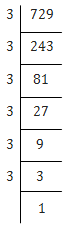729 = 3×3×3×3×3×3×1
⇒ 729 = (3×3)×(3×3)×(3×3)
⇒ 729 = (3×3×3)×(3×3×3)
⇒ 729 = (3×3×3)2
⇒ √729 = 3×3×3 = 27

(ii)400 = 2×2×2×2×5×5×1
⇒ 400 = (2×2)×(2×2)×(5×5)
⇒ 400 = (2×2×5)×(2×2×5)
⇒ 400 = (2×2×5)2
⇒ √400 = 2×2×5 = 20

(iii)1764 = 2×2×3×3×7×7
⇒ 1764 = (2×2)×(3×3)×(7×7)
⇒ 1764 = (2×3×7)×(2×3×7)
⇒ 1764 = (2×3×7)2
⇒ √1764 = 2 ×3×7 = 42

(iv)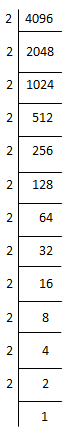4096 = 2×2×2×2×2×2×2×2×2×2×2×2
⇒ 4096 = (2×2)×(2×2)×(2×2)×(2×2)×(2×2)×(2×2)
⇒ 4096 = (2×2×2×2×2×2)×(2×2×2×2×2×2)
⇒ 4096 = (2×2×2×2×2×2)2
⇒ √4096 = 2×2×2 ×2×2×2 = 64

(v)7744 = 2×2×2×2×2×2×11×11×1
⇒ 7744 = (2×2)×(2×2)×(2×2)×(11×11)
⇒ 7744 = (2×2×2×11)×(2×2×2×11)
⇒ 7744 = (2×2×2×11)2
⇒ √7744 = 2×2×2×11 = 88

(vi)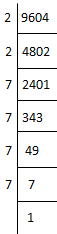9604 = 62 × 2 × 7 × 7 × 7 × 7
⇒ 9604 = ( 2 × 2 ) × ( 7 × 7 ) × ( 7 × 7 )
⇒ 9604 = ( 2 × 7 ×7 ) × ( 2 × 7 ×7 )
⇒ 9604 = ( 2×7×7 )2
⇒ √9604 = 2×7×7
= 98

(vii)5929 = 7×7×11×11
⇒ 5929 = (7×7)×(11×11)
⇒ 5929 = (7×11)×(7×11)
⇒ 5929 = (7×11)2
⇒ √5929 = 7×11 = 77

(viii)9216 = 2×2×2×2×2×2×2×2×2×2×3×3×1
⇒ 9216 = (2×2)×(2×2) × ( 2 × 2 ) × ( 2 × 2 ) × ( 2 × 2 ) × ( 3 × 3 )
⇒ 9216  =  ( 2 × 2 × 2 × 2 × 2 × 3)  × ( 2 × 2 × 2 × 2 × 2 × 3)
⇒ 9216 =   96 × 96
⇒ 9216 = ( 96 )2
⇒ √9216 = 96

(ix)529 = 23×23
⇒ 529 = (23)2
⇒ √529 = 23

(x)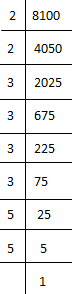8100 = 2×2×3×3×3×3×5×5×1
⇒ 8100 = (2×2) ×(3×3)×(3×3)×(5×5)
⇒ 8100 = (2×3×3×5)×(2×3×3×5)
⇒ 8100 = 90×90
⇒ 8100 = (90)2
⇒ √8100 = 90

5.For each of the following numbers, find the smallest whole number by which it should be multiplied so as to get a perfect square number. Also find the square root of the square number so obtained.
(i) 252
(ii) 180
(iii) 1008
(iv) 2028
(v) 1458
(vi) 768

Solution

(i)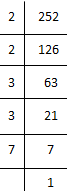252 = 2×2×3×3×7
= (2×2)×(3×3)×7
Here, 7 cannot be paired.
∴ We will multiply 252 by 7 to get perfect square.
New number = 252 × 7 = 17641764 = 2×2×3×3×7×7
⇒ 1764 = (2×2)×(3×3)×(7×7)
⇒ 1764 = 22×32×72
⇒ 1764 = (2×3×7)2
⇒ √1764 = 2×3×7 = 42

(ii)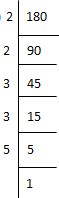180 = 2×2×3×3×5
= (2×2)×(3×3)×5
Here, 5 cannot be paired.
∴ We will multiply 180 by 5 to get perfect square.
New number = 180 × 5 = 900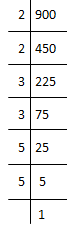900 = 2×2×3×3×5×5×1
⇒ 900 = (2×2)×(3×3)×(5×5)
⇒ 900 = 22×32×52
⇒ 900 = (2×3×5)2
⇒ √900 = 2×3×5 = 30

(iii)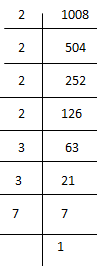1008 =  2×2×2×2×3×3×7
= (2×2)×(2×2)×(3×3)×7
Here, 7 cannot be paired.
∴ We will multiply 1008 by 7 to get perfect square.
New number = 1008×7 = 7056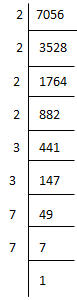7056 = 2×2×2×2×3×3×7×7
⇒ 7056 = (2×2)×(2×2)×(3×3)×(7×7)
⇒ 7056 = 22×22×32×72
⇒ 7056 = (2×2×3×7)2
⇒ √7056 = 2×2×3×7 = 84

(iv)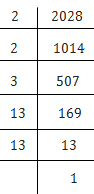2028 = 2×2×3×13×13
= (2×2)×(13×13)×3
Here, 3 cannot be paired.
∴ We will multiply 2028 by 3 to get perfect square.
New number = 2028×3 = 60846084 = 2×2×3×3×13×13
⇒ 6084 = (2×2)×(3×3)×(13×13)
⇒ 6084 = 22×32×132
⇒ 6084 = (2×3×13)2
⇒ √6084 = 2×3×13 = 78

(v)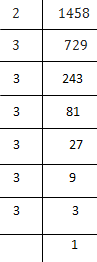1458 = 2×3×3×3×3×3×3
= (3×3)×(3×3)×(3×3)×2
Here, 3 cannot be paired.
∴ We will multiply 1458 by  2 to get perfect square.
New number = 1458 × 2 = 29162916 = 2×2×3×3×3×3×3×3
⇒ 2916 = (3×3)×(3×3)×(3×3)×(2×2)
⇒ 2916 =  32×32×32×22
⇒ 2916 = (3×3×3×2)2
⇒ √2916 = 3×3×3×2 = 54

(vi)768 = 2×2×2×2×2×2×2×2×3
= (2×2)×(2×2)×(2×2)×(2×2)×3
Here, 3 cannot be paired.
∴ We will multiply 768 by 3 to get perfect square.
New number = 768×3 = 23042304 = 2×2×2×2×2×2×2×2×3×3
⇒ 2304 = (2×2)×(2×2)×(2×2)×(2×2)×(3×3)
⇒ 2304 = 22×22×22×22×32
⇒ 2304 = (2×2×2×2×3)2
⇒ √2304 = 2×2×2×2×3 = 48

6. For each of the following numbers, find the smallest whole number by which it should be divided so as to get a perfect square. Also find the square root of the square number so obtained.
(i) 252
(ii) 2925
(iii) 396
(iv) 2645
(v) 2800
(vi) 1620

Solution

(i)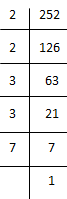252 = 2×2×3×3×7
= (2×2)×(3×3)×7
Here, 7 cannot be paired.
∴ We will divide 252 by 7 to get perfect square.
New number = 252 ÷ 7 = 36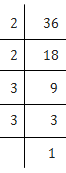36 = 2×2×3×3
⇒ 36 = (2×2)×(3×3)
⇒ 36 = 22×32
⇒ 36 = (2×3)2
⇒ √36 = 2×3 = 6

(ii)2925 = 3×3×5×5×13
= (3×3)×(5×5)×13
Here, 13 cannot be paired.
∴ We will divide 2925 by 13 to get perfect square.
New number = 2925 ÷ 13 = 225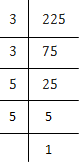225 = 3×3×5×5
⇒ 225 = (3×3)×(5×5)
⇒ 225 = 32×52
⇒ 225 = (3×5)2
⇒ √36 = 3×5 = 15

(iii)396 = 2×2×3×3×11
= (2×2)×(3×3)×11
Here, 11 cannot be paired.
∴ We will divide 396 by 11 to get perfect square.
New number = 396 ÷ 11 = 3636 = 2×2×3×3
⇒ 36 = (2×2)×(3×3)
⇒ 36 = 22×32
⇒ 36 = (2×3)2
⇒ √36 = 2×3 = 6

(iv)2645 = 5×23×23
⇒ 2645 = (23×23)×5
Here, 5 cannot be paired.
∴ We will divide 2645 by 5 to get perfect square.
New number = 2645 ÷ 5 = 529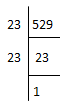529 = 23×23
⇒ 529 = (23)2
⇒ √529 = 23

(v)2800 = 2×2×2×2×5×5×7
= (2×2)×(2×2)×(5×5)×7
Here, 7 cannot be paired.
∴ We will divide 2800 by 7 to get perfect square.
New number = 2800 ÷ 7 = 400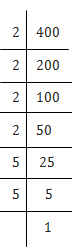400 = 2×2×2×2×5×5
⇒ 400 = (2×2)×(2×2)×(5×5)
⇒ 400 = (2×2×5)2
⇒ √400 = 20

(vi)
1620 =  2×2×3×3×3×3×5
= (2×2)×(3×3)×(3×3)×5
Here, 5 cannot be paired.
∴ We will divide 1620 by 5 to get perfect square.
New number = 1620 ÷ 5 = 324324 = 2×2×3×3×3×3
⇒ 324 = (2×2)×(3×3)×(3×3)
⇒ 324 = (2×3×3)2
⇒ √324 = 18

7. The students of Class VIII of a school donated Rs 2401 in all, for Prime Minister’s National Relief Fund. Each student donated as many rupees as the number of students in the class. Find the number of students in the class.

Solution

Let the number of students in the school be, x.
∴ Each student donate Rs.x .
Total many contributed by all the students = x×x = x2
Given, x2 = Rs.2401x2 = 7×7×7×7
⇒ x2  = (7×7)×(7×7)
⇒ x2 = 49×49
⇒ x =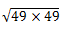⇒ x = 49
∴ The number of students = 49

8. 2025 plants are to be planted in a garden in such a way that each row contains as many plants as the number of rows. Find the number of rows and the number of plants in each row.

Solution

Let the number of rows be, x.
∴ the number of plants in each rows =  x.
Total many contributed by all the students = x×x = x2
Given,
x2 = Rs.2025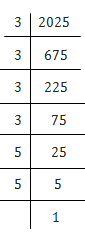x2 = 3×3×3×3×5×5
⇒ x2 = (3×3)×(3×3)×(5×5)
⇒ x2 = (3×3×5)×(3×3×5)
⇒ x2 = 45×45
⇒ x =⇒ x = 45
∴ The number of rows = 45 and the number of plants in each rows = 45.

9. Find the smallest square number that is divisible by each of the numbers 4, 9 and 10.

SolutionL.C.M of 4, 9 and 10 is (2×2×9×5) 180.

180 = 2×2×9×5
= (2×2)×3×3×5
= (2×2)×(3×3)×5
Here, 5 cannot be paired.
∴ we will multiply 180 by 5 to get perfect square.
Hence, the smallest square number divisible by 4, 9 and 10 = 180×5 = 900

10. Find the smallest square number that is divisible by each of the numbers 8, 15 and 20.

Solution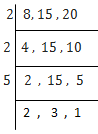L.C.M of 8, 15 and 20 is (2×2×5×2×3) 120.
120 = 2×2×3×5×2
= (2×2)×3×5×2
Here, 3, 5 and 2 cannot be paired.
∴ We will multiply 120 by (3×5×2) 30  to get perfect square.
Hence, the smallest square number divisible by 8, 15 and 20 =120×30 = 3600

Exercise 6.4

1.Find the square root of each of the following numbers by Division method.
(i) 2304
(ii) 4489
(iii) 3481
(iv) 529
(v) 3249
(vi) 1369
(vii) 5776
(viii) 7921
(ix) 576
(x) 1024
(xi) 3136
(xii) 900

Solution

(i)∴ √2304 = 48

(ii)∴ √4489 = 67

(iii)∴ √3481 = 59

(iv)∴ √529 = 29

(v)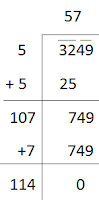∴  √3249 = 57

(vi)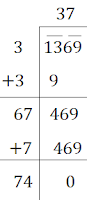∴ √1369 = 37

(vii)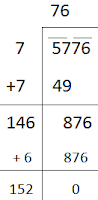∴ √5776 = 76

(viii)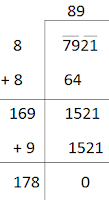∴ √7921 = 89

(ix)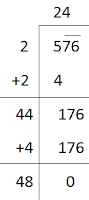∴ √576 = 24

(x)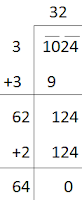∴ √1024 = 32

(xi)∴  √3136 = 56

(xii)∴ √900 = 30

2. Find the number of digits in the square root of each of the following numbers (without any calculation).
(i) 64
(ii) 144
(iii) 4489
(iv) 27225
(v) 390625

Solution

(i)∴  √64 = 8
Hence, the square root of the number 64 has 1 digit.

(ii)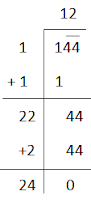∴  √144 = 12
Hence, the square root of the number 144 has 2 digits.

(iii)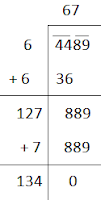∴ √4489 = 67
Hence, the square root of the number 4489 has 2 digits.

(iv)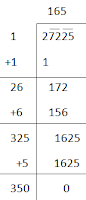∴ √27225 = 165
Hence, the square root of the number 27225 has 3 digits.

(v)∴  √390625 = 625
Hence, the square root of the number 390625 has 3 digits.

3. Find the square root of the following decimal numbers.
(i) 2.56
(ii) 7.29
(iii) 51.84
(iv) 42.25
(v) 31.36

Solution

(i)∴ √2.56 = 1.6

(ii)∴ √7.29 = 2.7

(iii)∴ √51.84 = 7.2

(iv)∴ √42.25 = 6.5

(v)∴ √31.36 = 5.6

4. Find the least number which must be subtracted from each of the following numbers so as to get a perfect square. Also find the square root of the perfect square so obtained.
(i) 402
(ii) 1989
(iii) 3250
(iv) 825
(v) 4000

Solution

(i)∴ We must subtracted 2 from 402 to get a perfect square.
New number = 402 – 2 = 400∴ √400 = 20

(ii)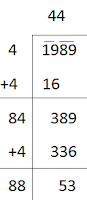∴ We must subtracted 53 from 1989 to get a perfect square.
New number = 1989 – 53 = 1936∴ √1936 = 44

(iii)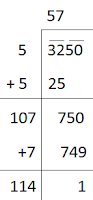∴ We must subtracted 1 from 3250 to get a perfect square.
New number = 3250 – 1 = 3249∴ √3249 = 57

(iv)∴ We must subtracted 41 from 825 to get a perfect square.
New number = 825 – 41 = 784∴ √784 = 28

(v)∴ We must subtracted 31 from 4000 to get a perfect square.
New number = 4000 – 31 = 3961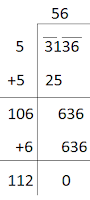∴ √3136 = 56

5. Find the least number which must be added to each of the following numbers so as to get a perfect square. Also find the square root of the perfect square so obtained.
(i) 525
(ii) 1750
(iii) 252
(iv) 1825
(v) 6412

Solution

(i)Here, (22) < 525 > (23)2
We can say 525 is ( 129 – 125 ) 4 less than (23)2.
∴ If we add 4 to 525, it will be perfect square.
New number = 525 + 4 = 529∴ √529 = 23

(ii)Here, (41)2 < 1750 > (42)2
We can say 1750 is ( 164 – 150 ) 14 less than (42)2.
∴ If we add 14 to 1750, it will be perfect square.
New number = 1750 + 14 = 1764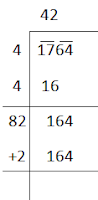∴√1764 = 42

(iii)Here, (15) < 252 > (16)2
We can say 252 is ( 156 – 152 ) 4 less than (16)2.
∴ If we add 4 to 252, it will be perfect square.
New number = 252 + 4 = 256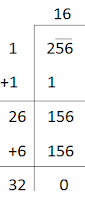∴ √256 = 16

(iv)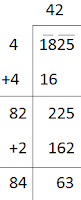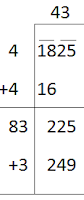Here, (42) < 1825 > (43)2
We can say 1825 is ( 249 – 225 ) 24 less than (43)2.
∴ If we add 24 to 1825, it will be perfect square.
New number = 1825 + 24 = 1849∴ √1849 = 43

(v)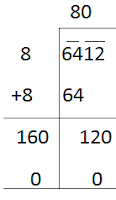Here, (80) < 6412 > (81)2
We can say 6412 is ( 161 – 12 ) 149 less than (81)2.
∴ If we add 149 to 6412, it will be perfect square.
New number = 6412 + 149 = 6561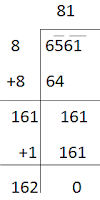∴ √6561 = 81

6. Find the length of the side of a square whose area is 441 m2.

Solution

Let the length of each side of the field = a
Then, area of the field = 441 m2
⇒ a2 = 441  m2
⇒a = √441 m∴ The length of each side of the field = a m = 21 m.

7. In a right triangle ABC, ∠B = 90°.
(a) If AB = 6 cm, BC = 8 cm, find AC
(b) If AC = 13 cm, BC = 5 cm, find AB

Solution

(a)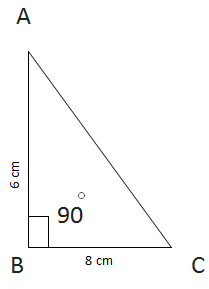Given, AB = 6 cm,
BC = 8 cm
Let AC be x cm.
∴ AC2 = AB2 + BC2Hence, AC = 10 cm.

(b)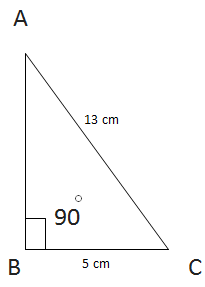Given, AC = 13 cm,
BC = 5 cm
Let AB be x cm.
∴ AC= AB+ BC2
⇒ AC- BC= AB2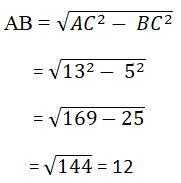Hence, AB = 12 cm

8. A gardener has 1000 plants. He wants to plant these in such a way that the number of rows and the number of columns remain same. Find the minimum number of plants he needs more for this.

Solution

Let the number of rows and column be, x.
∴ Total number of row and column= x×x = x2
As per question, x2 = 1000
⇒ x = √1000Here, (31)2 < 1000 > (32)2
We can say 1000 is ( 124 – 100 ) 24 less than (32)2.
∴ 24 more plants is needed.

9. There are 500 children in a school. For a P.T. drill they have to stand in such a manner that the number of rows is equal to number of columns. How many children would be left out in this arrangement.

Solution

Let the number of rows and column be, x.
∴ Total number of row and column= x × x = x2
As per question, x2 = 500
x = √500Hence, 16 children would be left out in the arrangement.

## NCERT Solutions for Class 8 Maths Chapter 6 Squares and Square Roots

Chapter 6 NCERT Solutions will help an individual to increase concentration and in this chapter we will be discussing about Squares and Square Roots. If a natural number m can be expressed as n2, where n is also a natural number, then m is a square number.

• If a number has 1 or 9 in the units place, then it’s square ends in 1.

• When a square number ends in 6, the number whose square it is, will have either 4 or 6 in unit’s place.

• There are 2n non perfect square numbers between the squares of the numbers n and (n + 1).

• If a natural number cannot be expressed as a sum of successive odd natural numbers starting with 1, then it is not a perfect square.

There are total 4 exercises in the Chapter 6 NCERT Solutions. Below you will also find exercisewise NCERT Solutions of every question will develop your skills and improving marks in the examinations.

Subject matter experts of Studyrankers have prepared these NCERT Solutions for Class 8 by keeping in the mind about the needs of students so they can clear their doubts.

### NCERT Solutions for Class 8 Maths Chapters:

 Chapter 1 Rational Numbers Chapter 2 Linear Equations in Variable Chapter 3 Understanding Quadrilaterals Chapter 4 Practical Geometry Chapter 5 Data Handling Chapter 7 Cube and Cube Roots Chapter 8 Comparing Quantities Chapter 9 Algebraic Expressions and Identities Chapter 10 Visualizing Solid Shapes Chapter 11 Mensuration Chapter 12 Exponents and Powers Chapter 13 Direct and Inverse Proportions Chapter 14 Factorization Chapter 15 Introduction to Graphs Chapter 16 Playing with Numbers

FAQ on Chapter 6 Squares and Square Roots

#### How many exercises in Chapter 6 Squares and Square Roots?

Chapter 6 NCERT Solutions consist of four exercises which is going to help you a lot in the preparation of examinations. There are variety of concepts given in this chapter 6 Class 8 Maths textbook that will develop your necessary skill to solve more and more questions.

#### Express 169 as the sum of first 13 odd numbers.

We have 169 = 132
= Sum of first 13 odd numbers
= 1 + 3 + 5 + 7 + 9 + 11 + 13 + 15 + 17 + 19 + 21 + 23 + 25
= (1 + 25) + (3 + 23) + (5 + 21) + (7 + 19) + (9 + 17) + (11 + 15) + 13
= 26 + 26 + 26 + 26 + 26 + 26 + 13
= (6 × 26) + 13 = 156 + 13 = 169.

#### What will be the unit’s digit in the square of 1251?

1251 is 1 (as 12 = 1. 1 in the unit’s place).

#### How many numbers lie between squares of 16 and 17?

Between 16 and 17, there are 2 × 16, i.e. 32 numbers.